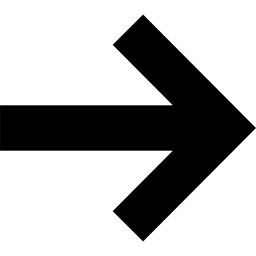## Learn to Use ITensor

main / book / index C++v3 | C++v2 | Julia

# Index Objects

The most basic element of ITensor is not actually a tensor: it is a tensor index, an object of type Index. (By tensor index we mean i,j, or k in an expression like $T_{ijk}$ .)

ITensors are "intelligent tensors" because they "know" what indices they have. This is possible since an Index carries extra information beyond its dimension.

The simplest way to construct an Index is to give its dimension:

auto i = Index(3);


Upon creation, an Index gets "stamped" with a permanent ID number that allows copies of the Index to recognize each other. Typically you do not need to look at these ID numbers; it is enough to know that indices match (compare equal) if they are copies of the same original index:

auto j = i;  //make a copy of i
Print(j==i); //prints: j==i = true


(Also two indices must have the same tags and prime level to compare equal; see below).

To access the dimension, or size of an Index, use the dim function

println("The dimension of index i is ",dim(i));
//prints: The dimension of index i is 3

#include "itensor/all.h"
using namespace itensor;

int main()
{
auto i = Index(3);
println("The dimension of index i is ",dim(i));
return 0;
}


Certain properties of an Index cannot be changed, such as its dimension and ID number. However, a new Index can be created to replace an old one. The philosophy of ITensor is that indices have a meaning given at the time they are created.

### Tagging Indices

When constructing an Index you can additionally provide a comma-separated list of tags which help to identify this Index. Only Index objects with the exact same set of tags will compare equal to one other (when compared with the == operator). Tags are also useful for identifying certain indices or retrieving a certain index from an ITensor.

To specify tags when defining an Index, list them after the Index dimension:

auto i = Index(2,"i");
auto s1 = Index(3,"n=1,Site");


An Index can have up to four tags, and each tag can be a maximum of seven characters long. These limitations are to ensure your calculations run at maximum efficiency.

We will see how to modify tags later, in the chapter on Modifying Index Objects.

### Priming Indices

Sometimes it is necessary to temporarily distinguish two copies of the same Index from each other, such as when they are two indices of the same ITensor. A lightweight and convenient way to do this is to adjust the "prime level" of an Index. The prime level is an integer that starts out as zero and can be set, or raised and lowered to other integer values. Only two copies of the same Index with the same prime level will compare equal to each other.

Calling prime(i) will produce a copy of i with prime level increased by 1. Because this copy has a different prime level from the original Index i, it will no longer compare equal to i.

auto i1 = prime(i);

println("The prime level of i1 is ",primeLevel(i1));
//prints: The prime level of i1 is 1

printfln("i1==i is %s",i1==i);
//prints: i1==i is false


There are many convenient ways to manipulate Index prime levels. The prime function accepts an optional increment amount:

auto i3 = prime(i,3);

println(primeLevel(i3));
//prints: 3


Calling noPrime resets the prime level to zero.

auto i0 = noPrime(i3);

println(primeLevel(i0));
//prints: 0


Note that the above names (i3, i0, etc.) are just to make the code easier to read—you can use any variable names you want regardless of the prime level.

We will see more ways to manipulate primes as we work with ITensors with multiple indices, and in the chapter on Modifying Indices.

### Printing Indices

Printing an Index shows useful information about it:

auto i = Index(3,"index i,Link");

println(i);


The output shows the dimension, id, and tags of i. The ID numbers are random 64 bit integers which vary each time you construct a new index (even if it has the same dimension and tags each time). For reasons of space and readability, only a portion of the ID is shown.

The prime level is displayed at the end:

println(prime(i,2));

println(prime(i,10));


### Summary

The indices of an ITensor are Index objects. An Index has a fixed dimension and an ID number that are set when it is constructed; copies of that Index will always have the same dimension and ID.

Index objects can also carry tags and a prime level. Tags and prime levels can be useful in various situations, such as retrieving an Index from an ITensor. The tags and prime level of an Index can be modified.

For a complete listing of all of the methods of class Index, view the detailed documentation.ITensor Library OverviewITensor BasicsBack to BookBack to Main